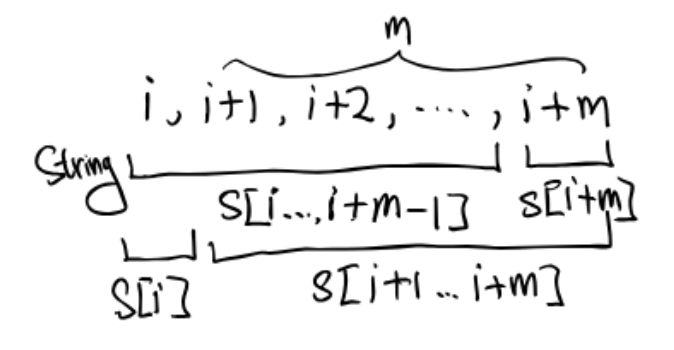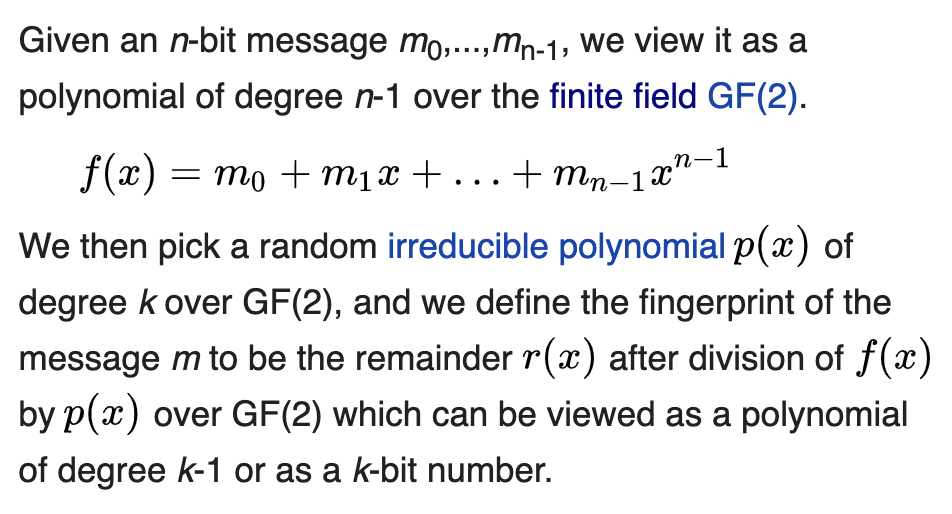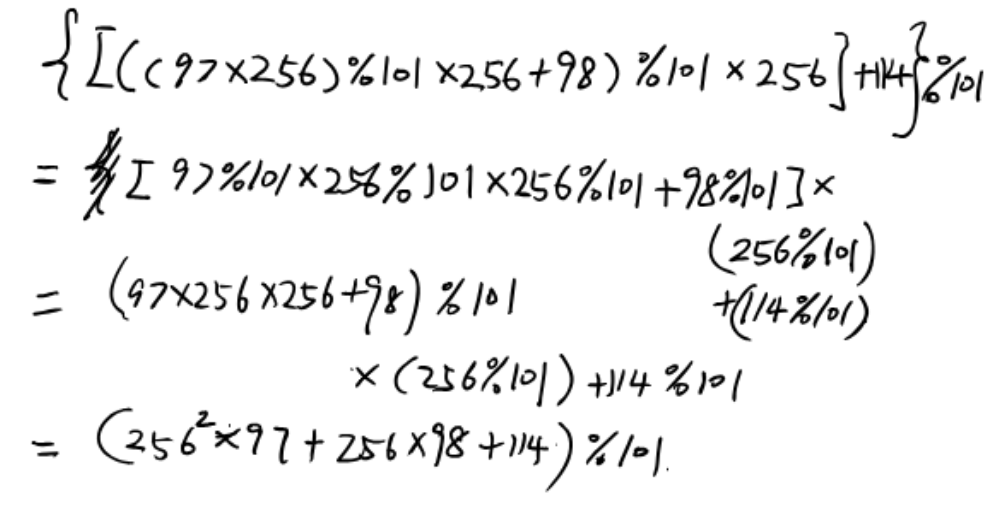Reference: Wikipedia

## Brute-force Substring Matching

This algorithm works well in many practical cases, but can exhibit relatively long running times in certain examples, such as searching for a pattern string of 10,000 “a”s followed by a single “b” in a search string of 10 million “a”s, in which case it exhibits its worst-case $O(nm)$ time.

• The Knuth-Morris-Pratt algorithm reduces this to $O(n)$ using pre-computation to examine each text character only once. (wiki link (nice explanation))
• The Boyer-Moore algorithm skips forward not by 1 character, but by as many as possible for the search to succeed. It focuses on the outer loop.

The Rabin-Karp algorithm focuses on the inner loop.

## Rabin-Karp algorithm

This algorithm uses hashing to find any one of a set of pattern strings a text. For text of length $n$ and $p$ patterns of combined length $m$, its average and best case running time is $O(n + m)$ in space $O(p)$, but its worst-case time is $O(nm)$.

In contrast, the Aho–Corasick string-matching algorithm has asymptotic worst-time complexity $O(n+m)$ in space $O(m)$.

A practical application of the algorithm is detecting plagiarism.

Rather than pursuing more sophisticated skipping, the Rabin–Karp algorithm seeks to speed up the testing of equality of the pattern to the substrings in the text by using a hash function.

The algorithm exploits the fact that if two strings are equal, their hash values are also equal. Thus, string matching is reduced (almost) to computing the hash value of the search pattern and then looking for substrings of the input string with that hash value.

One problem is that because there are so many different strings and so few hash values, some differing strings will have the same hash value. If the hash values match, the pattern and the substring may not match; consequently, the potential match of search pattern and the substring must be confirmed by comparing them; that comparison can take a long time for long substrings.

Luckily, a good hash function on reasonable strings usually does not have many collisions, so the expected search time will be acceptable.

The algorithm is as shown:

Runtime analysis:

Naively computing the hash value for an m-length string requires $O(m)$ time because each character is examined.

Lines A, B, and D each require $O(m)$ time. However, Line A is only executed once, and Line D is only executed if the hash values match, which is unlikely to happen more than a few times.

Line C is executed $O(n)$ times, but each comparison only requires constant time, so its impact is $O(n)$. The issue is Line B: stringHash := hash(s[i..i+m-1])

So, with the naive method of computing the hash value of the substring on each loop, the algorithm requires $O(nm)$ time, the same complexity as the aforementioned brute-force method.

Rolling Hash

For speed, the hash must be computed in constant time. The trick is the variable stringHash already contains the previous hash value of s[i..i+m-1]. If that value can be used to compute the next hash value in constant time, then computing successive hash values will be fast.

The trick can be exploited using a rolling hash. A rolling hash is a hash function specially designed to enable this operation. A trivial (but not very good) rolling hash function just adds the values of each character in the substring. This rolling hash formula can compute the next hash value from the previous value in constant time:

s[i+1..i+m] = s[i..i+m-1] - s[i] + s[i+m]But this hashing method like other poor hash methods will result in poor performance in Line C (if patternHash = stringHash:) and it would be executed $O(n)$ times. And the following character-by-character comparison of strings with length $m$ takes $O(m)$ time, the whole algorithm then takes a worst-case $O(nm)$ time.

### Rabin fingerprint

The key to the Rabin–Karp algorithm’s performance is the efficient computation of hash values of the successive substrings of the text. The Rabin fingerprint is a popular and effective rolling hash function.

The hash function described here is not a Rabin fingerprint, but it works equally well. It treats every substring as a number in some base, the base being usually the size of the character set.For example, if the substring is “hi”, the base is 256, and prime modules is 101, then the hash value would be:

For example (hash rolling), if we have text “abracadabra” and we are searching for a pattern of length 3, the hash of the first substring “abr”, using 256 as the base and 101 as the prime modulus is:$$(x + y) \bmod{z} = \left[ x \bmod{z} + y \bmod{z} \right] \bmod{z}$$
$$(x \times y) \bmod{z} = \left[(x \bmod{z}) \times (y\bmod{z})\right] \bmod{z}$$

Then we compute the hash of the next substring, “bra”, from the hash “abr” by subtracting the number added for the first ‘a’, i.e. $97 \times 256^2$, multiplying by the base and adding for the last ‘a’ of “bra”, i.e. $97 \times 256^0$.

Although ((256 % 101) x 256) % 101 is the same as 256^2 % 101, to avoid overflowing integer maximums when the pattern string is longer (e.g. ‘Rabin-Karp’ is 10 characters, 256^9 is the offset without modulation), the pattern length base offset is pre-calculated in a loop, modulating the result each iteration.

If the substrings in question are long, this algorithm achieves great savings compared with many other hashing schemes.

The Rabin-Karp algorithm is an algorithm of choice for multiple pattern search.

To find any of a large number, say $k$, fixed length patterns in a text, a simple variant of the algorithm uses a Bloom filter or a set data structure to check whether the hash of a given string belongs to a set of hash values of patterns we are looking for:

We assume all the substrings have a fixed length $m$.

A naïve way to search for $k$ patterns is to repeat a single-pattern search taking $O(n+m)$ time, totaling in $O((n+m)k)$ time. In contrast, the above algorithm above can find all k patterns in $O(n+km)$ expected time, assuming that a hash table check works in $O(1)$ expected time. A deterministic $O(n+km)$ solution is the Aho–Corasick algorithm.

## KMP Implementation

CommentJunhao Wang
Hi, I was a master student at USC studying Computer Science.